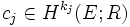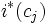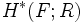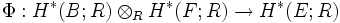# Leray-Hirsch theorem for cohomology

You might be looking for: Leray-Hirsch theorem for K-theory

## Statement

Let$p:E \to B$ be a fiber bundle with fiber space$F$, and$R$ be a commutative unital ring, such that the following hold:

•$H^n(F;R)$ is a finitely generated free$R$-module for every$n$
• There exist classes$c_j \in H^{k_j}(E;R)$ whose restrictions$i^*(c_j)$ form a basis for$H^*(F;R)$ in each fiber$F$, via the inclusion of the fiber in$E$ (the choice of these classes needs to be made independent of the fiber)

Then the map:$\Phi: H^*(B;R) \otimes_R H^*(F;R) \to H^*(E;R)$

given by:$\sum_{jk} b_j \otimes i^*(c_k) \mapsto \sum_{jk} p^*(b_j) \smile c_j$

is an isomorphism.